﻿ 粘弹性自由阻尼加筋板的随机响应分析和试验研究
 舰船科学技术2020, Vol. 42Issue (1): 62-67PDF

1. 上海交通大学 海洋工程国家重点实验室，上海 200240;
2. 高新船舶与深海开发装备协同创新中心，上海 200240

Random response analysis and experimental study of stiffened plate with unconstrained damped layer
CHEN Wei1,2, XIA Li-juan1,2
1. State Key Laboratory of Ocean Engineering, Shanghai Jiaotong University, Shanghai 200240, China;
2. Collaborative Innovation Center for Advanced Ship and Deep-Sea Exploration, Shanghai 200240, China
Abstract: For viscoelastic unconstrained damped structures, the modal loss factor correction formula considering both the viscoelastic damping loss factor and the elastic modulus frequency change effect is derived based on the modal strain energy method, and a ribbed plate is selected for calculation and verification. Random response experiment was carried out for a ship damping stiffened plate. The modal loss factor and random response of the model were numerically calculated and compared. The results show that it is feasible to determine the modal loss factor of unconstrained damped structures with the modified formula and calculate the random response. The method has reference value for the correlation analysis of vibration damping.
Key words: viscoelastic damping     modal loss factor     correction formula     random response
0 引　言

1 理论分析及修正公式推导 1.1 模态应变能法基本原理

 $M\ddot x + Kx = 0{\text{，}}$ (1)

 $K = {K_R} + i{K_I}{\text{，}}$ (2)
 ${K_R}{\rm{ = }}{K_e} + {K_{vR}}{\text{，}}$ (3)
 ${K_I} = {\eta _v} \cdot {K_{vR}}{\text{。}}$ (4)

 $X = {\phi ^{*(r)}}{e^{i\omega _r^*t}}{\text{，}}$ (5)

 ${\phi ^{*(r)}}{\rm{ = }}\phi _{^R}^{*(r)} + i\phi _I^{*(r)}{\text{，}}$ (6)
 $\omega _r^* = {\omega _r}\sqrt {1 + i{\xi ^{(r)}}} {\text{。}}$ (7)

 $\omega _r^2{\rm{(}}1 + i{\xi ^{(r)}}{\rm{) = }}\frac{{{\phi ^{*(r)T}}{K_R}{\phi ^{*(r)}}}}{{{\phi ^{*(r)T}}M{\phi ^{*(r)}}}} + i\frac{{{\phi ^{*(r)T}}{K_I}{\phi ^{*(r)}}}}{{{\phi ^{*(r)T}}M{\phi ^{*(r)}}}}{\text{。}}$ (8)

 $\begin{split} & {\xi ^{(r)}} = \frac{{{\phi ^{(r)T}}{K_I}{\phi ^{(r)}}}}{{{\phi ^{(r)T}}{K_R}{\phi ^{(r)}}}}{\rm{ = }}{\eta _v}\frac{{{\phi ^{(r)T}}{K_{vR}}{\phi ^{(r)}}}}{{{\phi ^{(r)T}}{K_R}{\phi ^{(r)}}}}{\rm{ = }}\\ & \quad \quad{\eta _v}\frac{{V_v^{(r)}}}{{{V^{(r)}}}}{\rm{ = }}{\eta _v}\frac{{V_v^{(r)}}}{{V_e^{(r)} + V_v^{(r)}}}{\rm{ = }}{\eta _v}{R^{(r)}}{\text{。}} \end{split}$ (9)

1.2 考虑粘弹性阻尼频变特性的模态损耗因子修正计算公式推导

 $R_{_{ref}}^{(r)} = \frac{{V_{vref}^{(r)}}}{{V_{eref}^{(r)} + V_{vref}^{(r)}}}{\text{，}}$ (10)

 ${R^{(r)}} = \frac{{V_v^{(r)}}}{{V_e^{(r)} + V_v^{(r)}}} = \frac{{\frac{E}{{{E_{ref}}}}V_{vref}^{(r)}}}{{V_{eref}^{(r)} + \frac{E}{{{E_{ref}}}}V_{vref}^{(r)}}} = \frac{{E'}}{{\frac{1}{{R_{ref}^{(r)}}} - 1 + E'}}{\text{。}}$ (11)

 ${\xi ^{(r)}}{\rm{ = }}{\eta _v}{R^{(r)}}{\rm{ = }}{\eta _v}({\omega _r}) \cdot {R^{(r)}}{\text{。}}$ (12)

 $\xi _{{\omega _r}}^{^{(r)}} = {\eta _v}({\omega _r}) \cdot \frac{{E'({\omega _r})}}{{\frac{1}{{R_{_{ref}}^{(r)}}} - 1 + E'({\omega _r})}}{\text{。}}$ (13)

2 粘弹性阻尼压筋板算例验证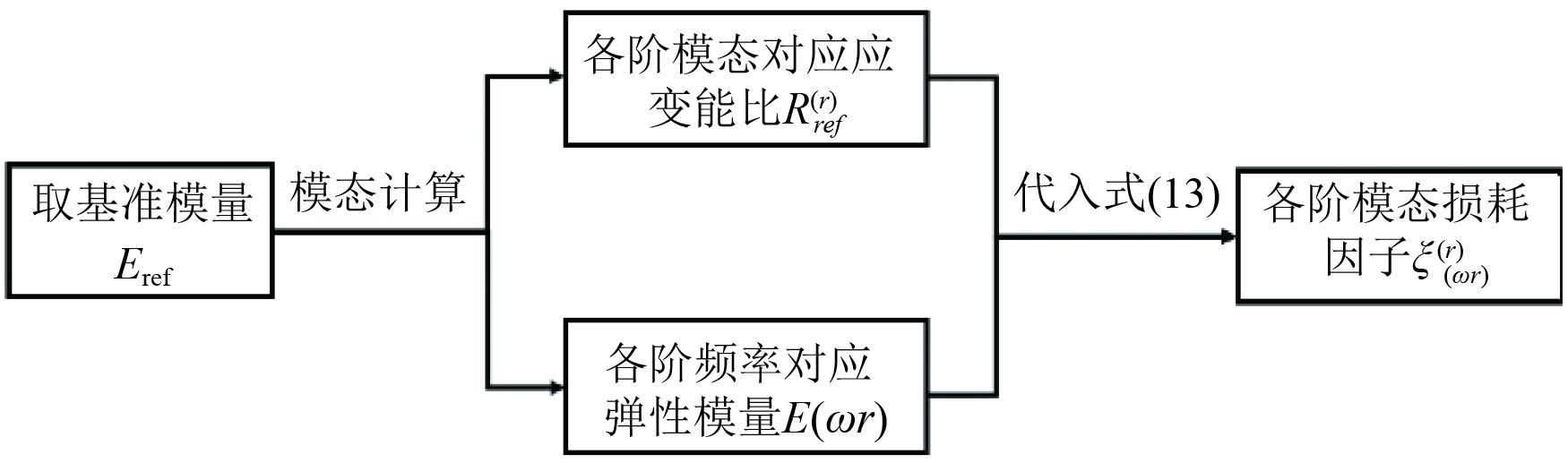图 1 采用修正公式计算模态损耗因子流程 Fig. 1 Calculating modal loss factor by correction formula

 ${\rm {Log}}E = 7.51 + \frac{{2.27{f^{0.79}}}}{{67.6 + {f^{0.79}}}}{\text{，}}$ (14)
 ${\rm {Log}}\eta = 0.23 - 0.1{\rm {Log}}f - 0.02\sqrt {1 + 25{{({\rm {Log}}f - 2.04)}^2}}{\text{。}}$ (15)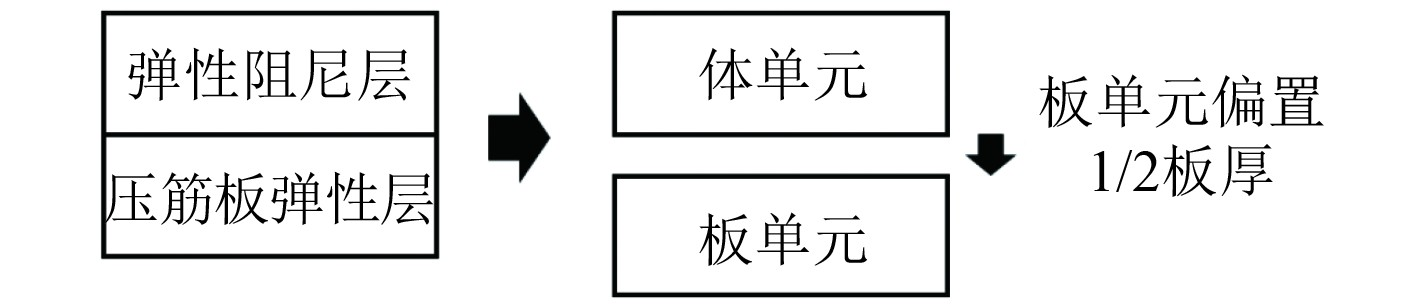图 2 压筋板有限元模拟方式 Fig. 2 Finite element simulation of ribbed plate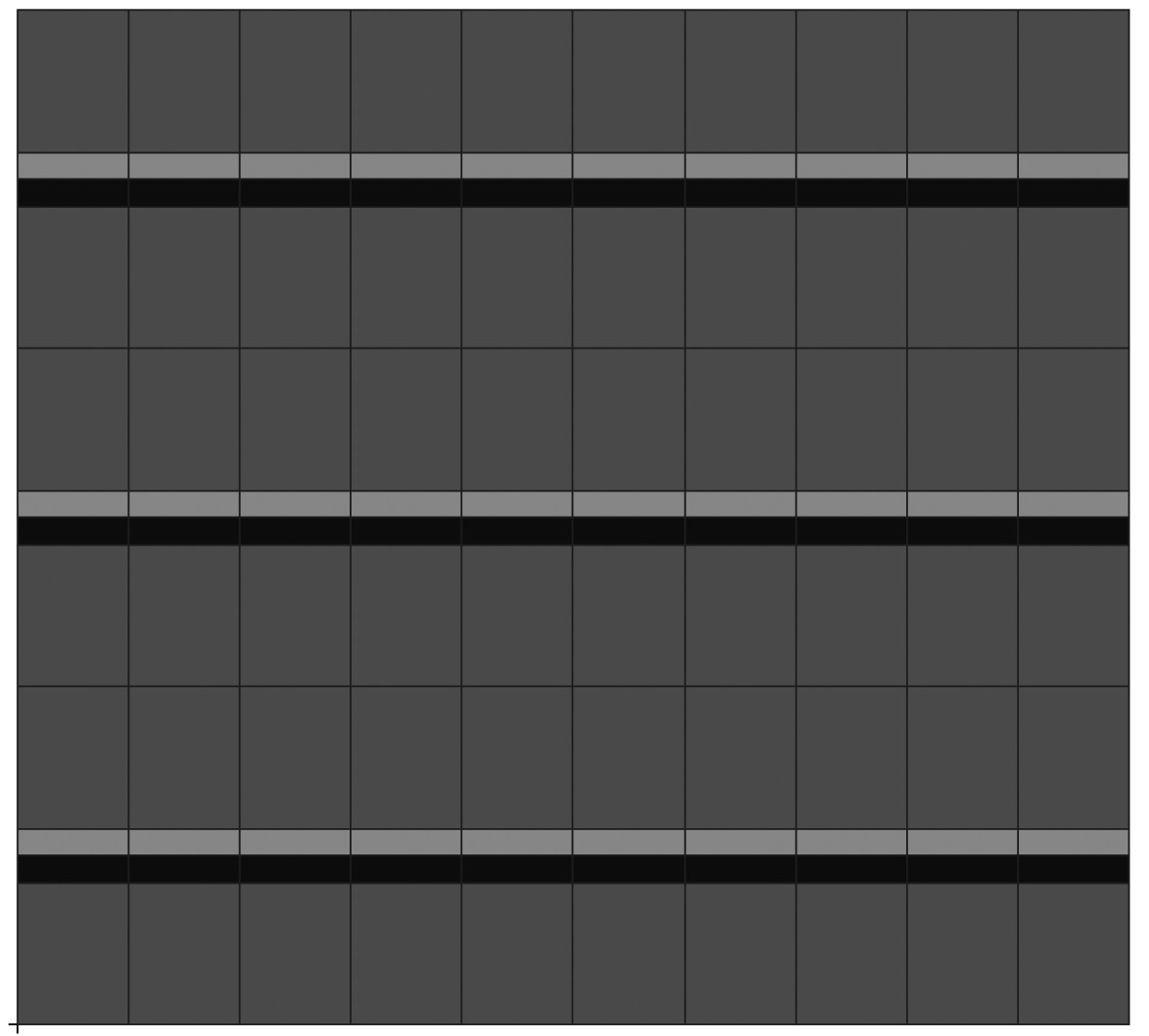图 3 压筋板有限元模型 Fig. 3 Finite element model of ribbed plate表 1 压筋板模态损耗因子的计算与试验对比 Tab.1 Comparison of calculation and experimental modal loss factor
3 考虑频变的粘弹性阻尼加筋板随机响应研究 3.1 随机响应简介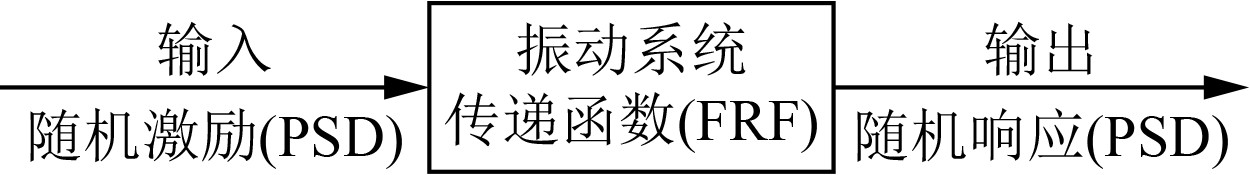图 4 随机响应示意图 Fig. 4 Random response diagram

 ${a_{1/3}} = \sqrt {\int_{{f_l}}^{{f_u}} {PSD{\rm d}f} } {\text{。}}$ (16)
 ${a_{mean}} = \sqrt {\sum {a_{1/3}^2} } {\text{。}}$ (17)表 2 1/3倍频程范围 Tab.2 Range of 1/3 octave
3.2 粘弹性阻尼加筋板随机响应试验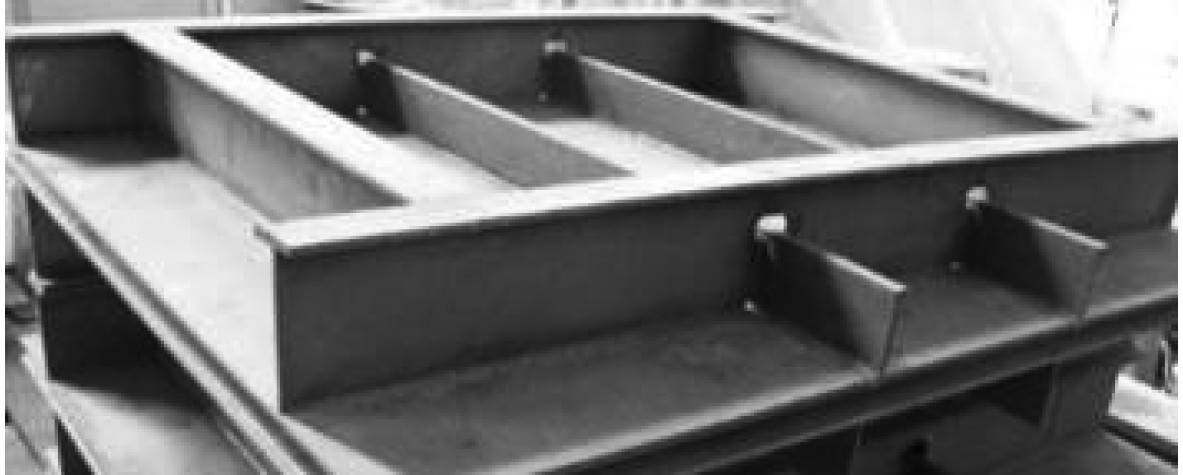图 5 船舶加筋板试验模型 Fig. 5 Model of ribbed plate in experiment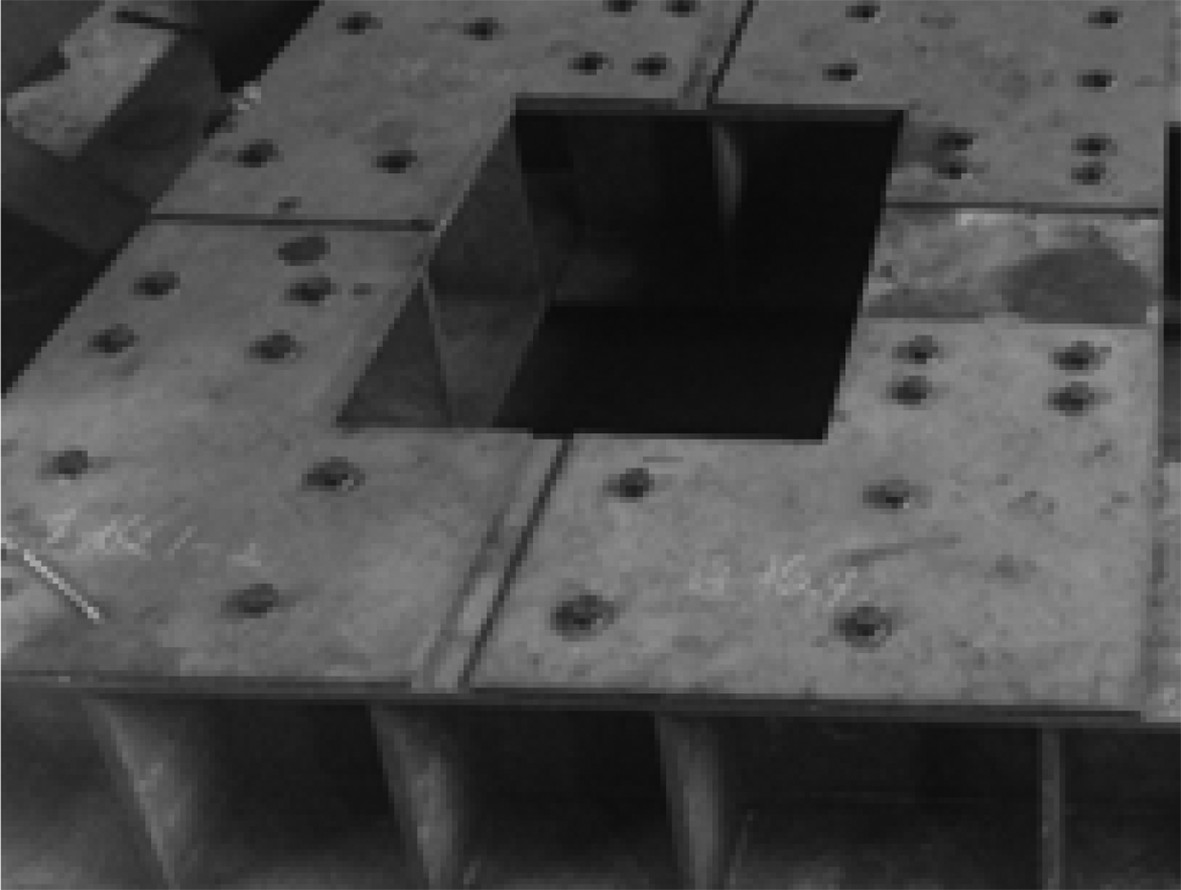图 6 试验支撑基座 Fig. 6 Support base in experiment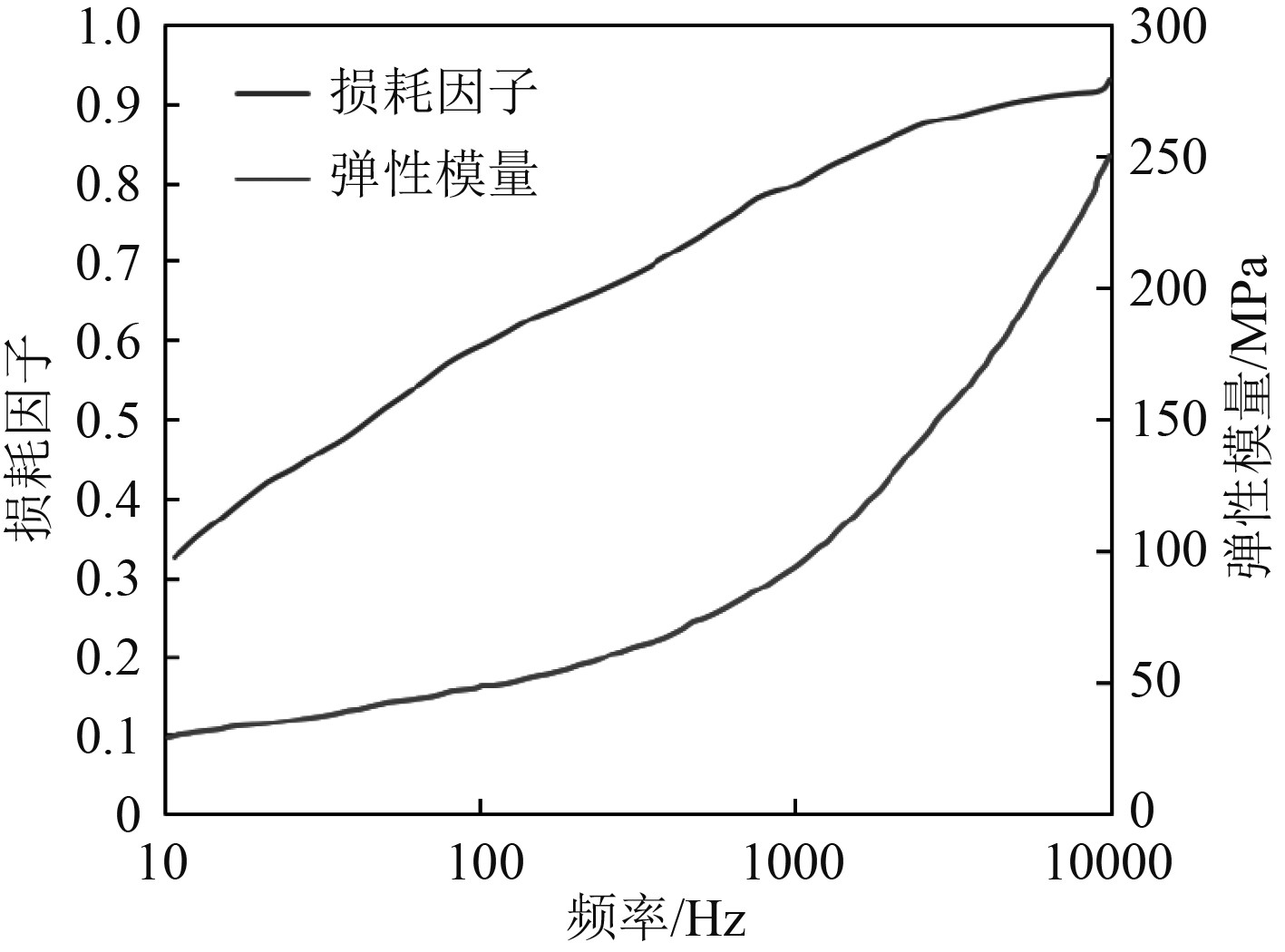图 7 丁基橡胶频变特性图 Fig. 7 Frequency change characteristic of butyl rubber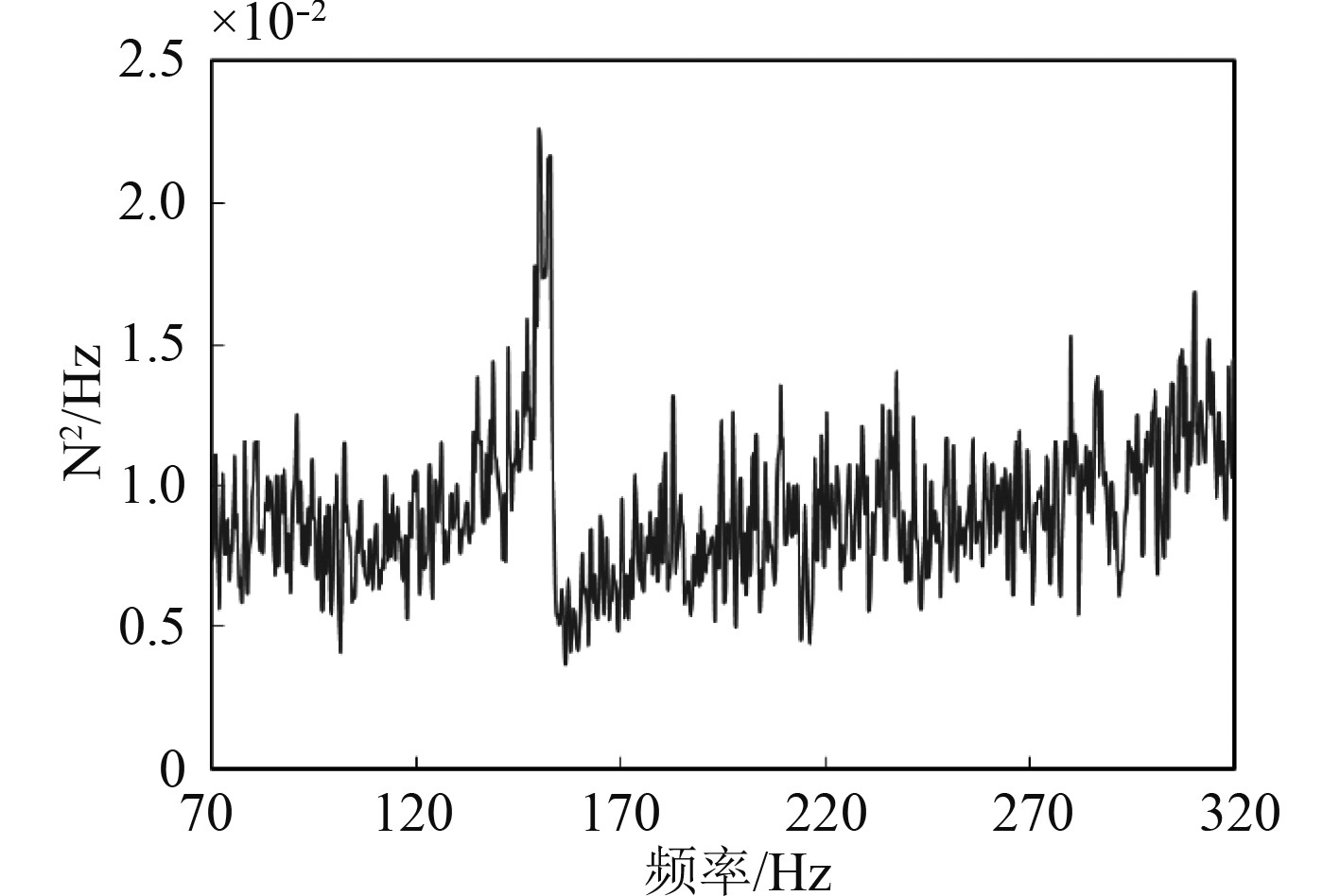图 8 随机激励PSD谱 Fig. 8 Random incentive PSD spectrum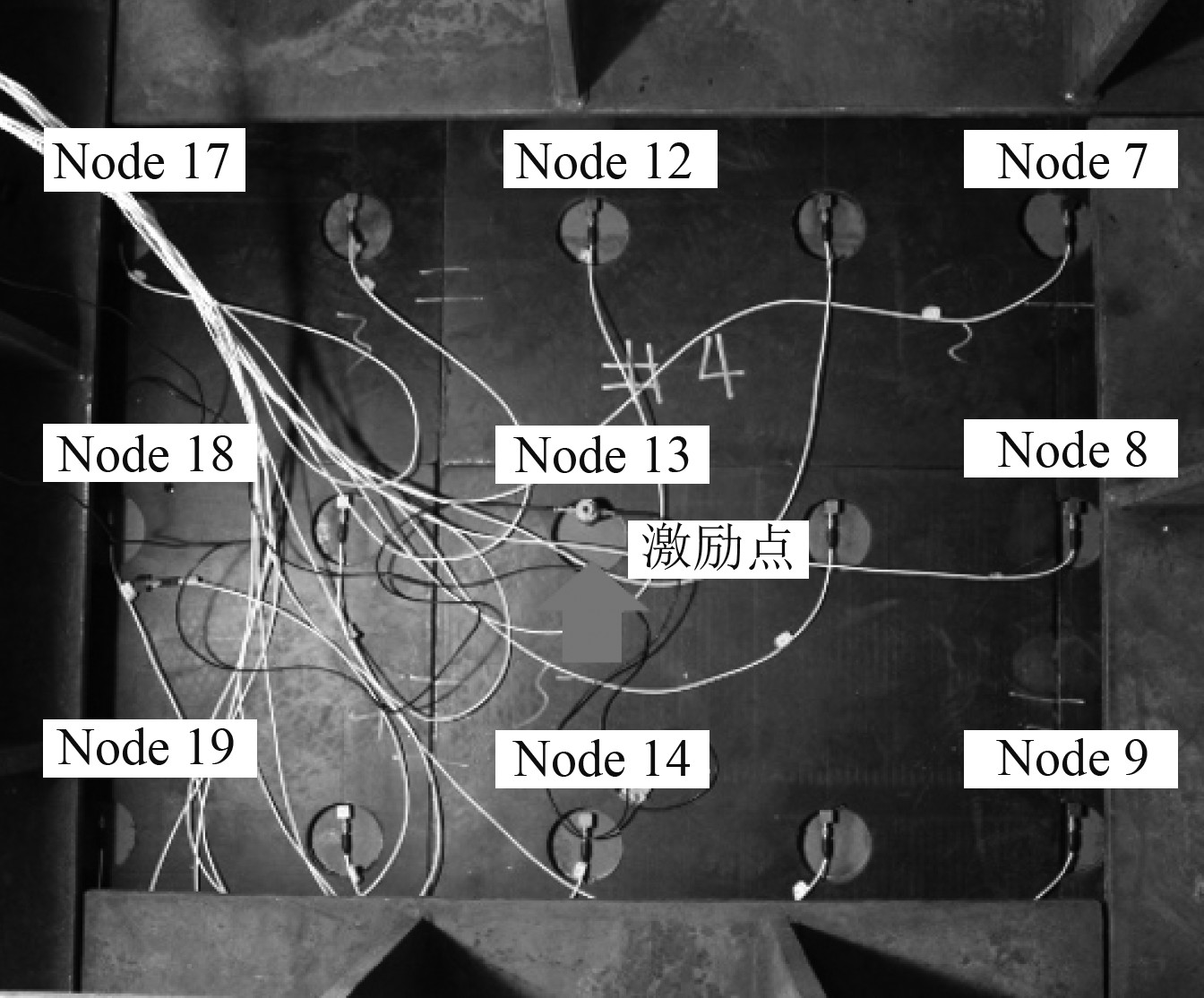图 9 试验测点分布 Fig. 9 Test nodes distribution
3.3 粘弹性阻尼加筋板随机响应计算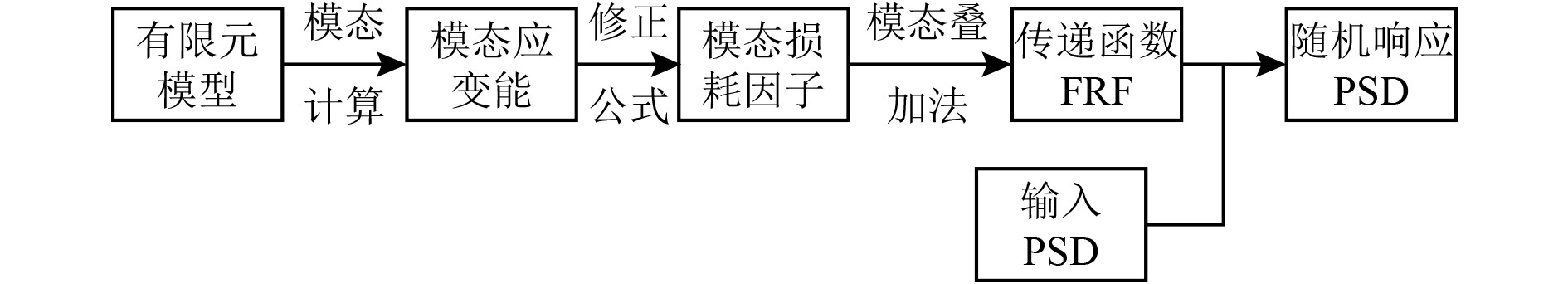图 10 随机响应计算流程 Fig. 10 Random response calculation process
 ${S_{yy}}(\omega ) = {\left| {H(\omega )} \right|^2}{S_{xx}}(\omega ){\text{。}}$ (18)表 3 阻尼加筋板模型的模态损耗因子计算值 Tab.3 Calculation modal loss factor of the model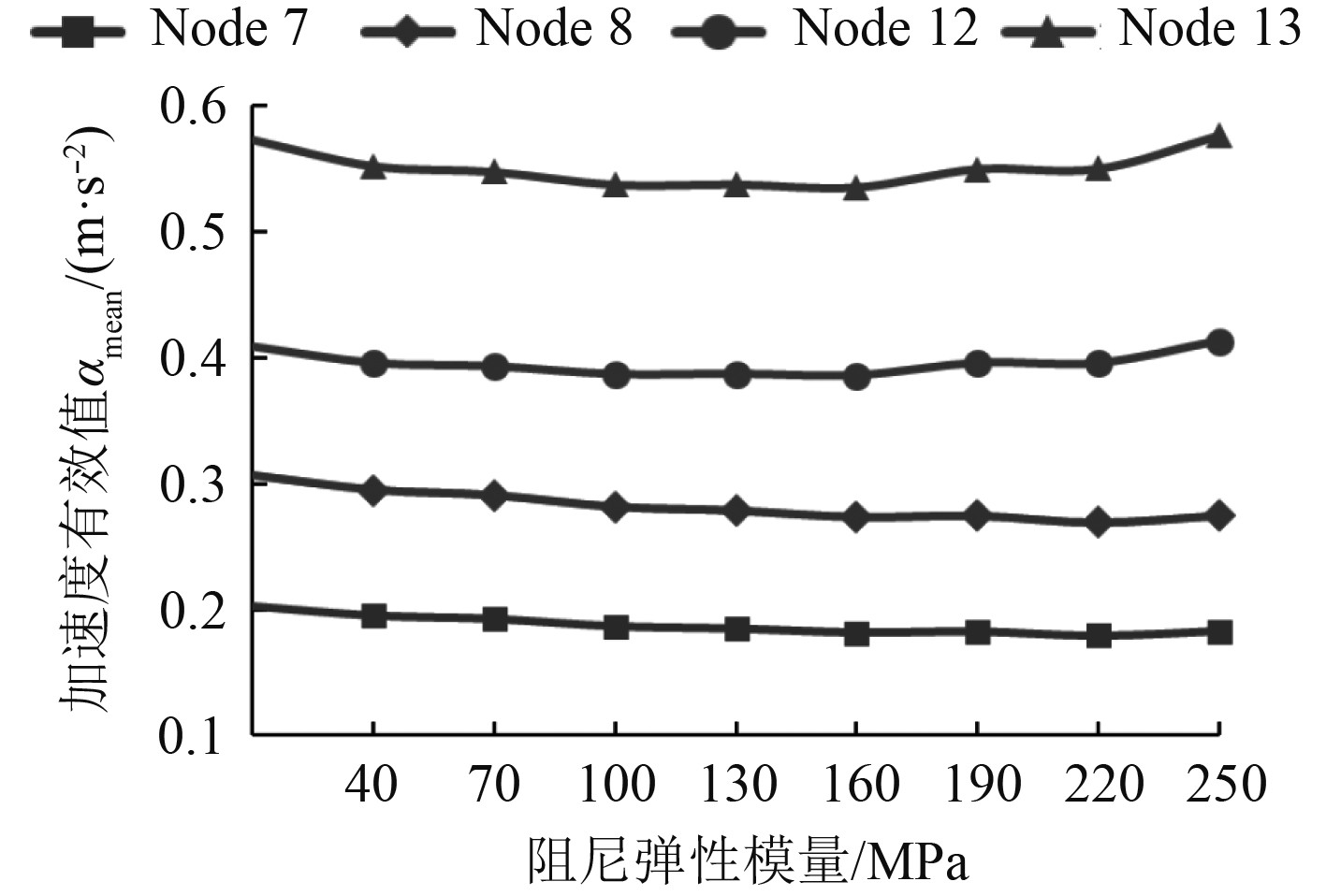图 11 加筋板加速度有效值随阻尼弹性模量变化图 Fig. 11 Acceleration RMS of stiffened plate with different damping elastic modulus表 4 阻尼加筋板模型的随机响应计算值和试验值对比 Tab.4 Comparison of calculation and experiment results of damped stiffened plate model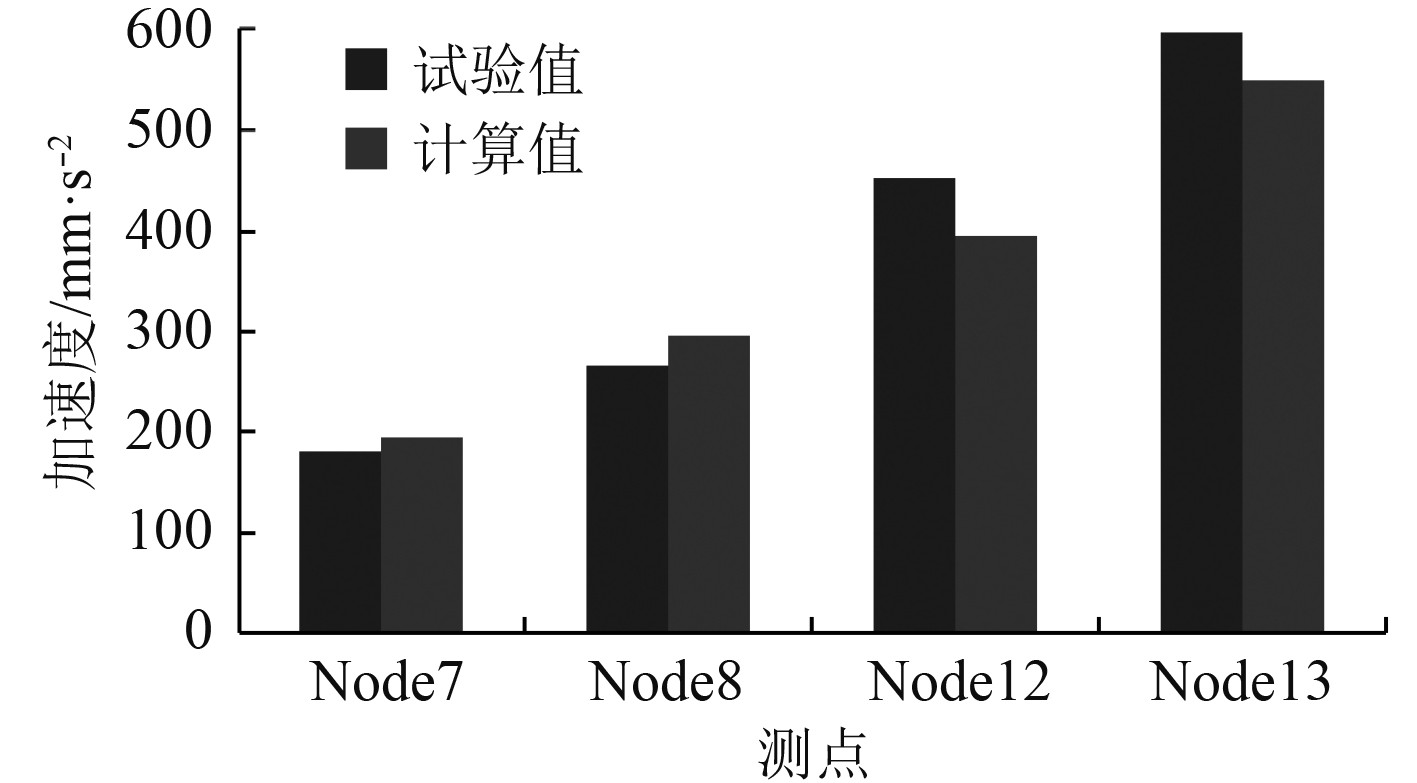图 12 各测点频率段加速度有效值amean Fig. 12 Acceleration RMS amean of each node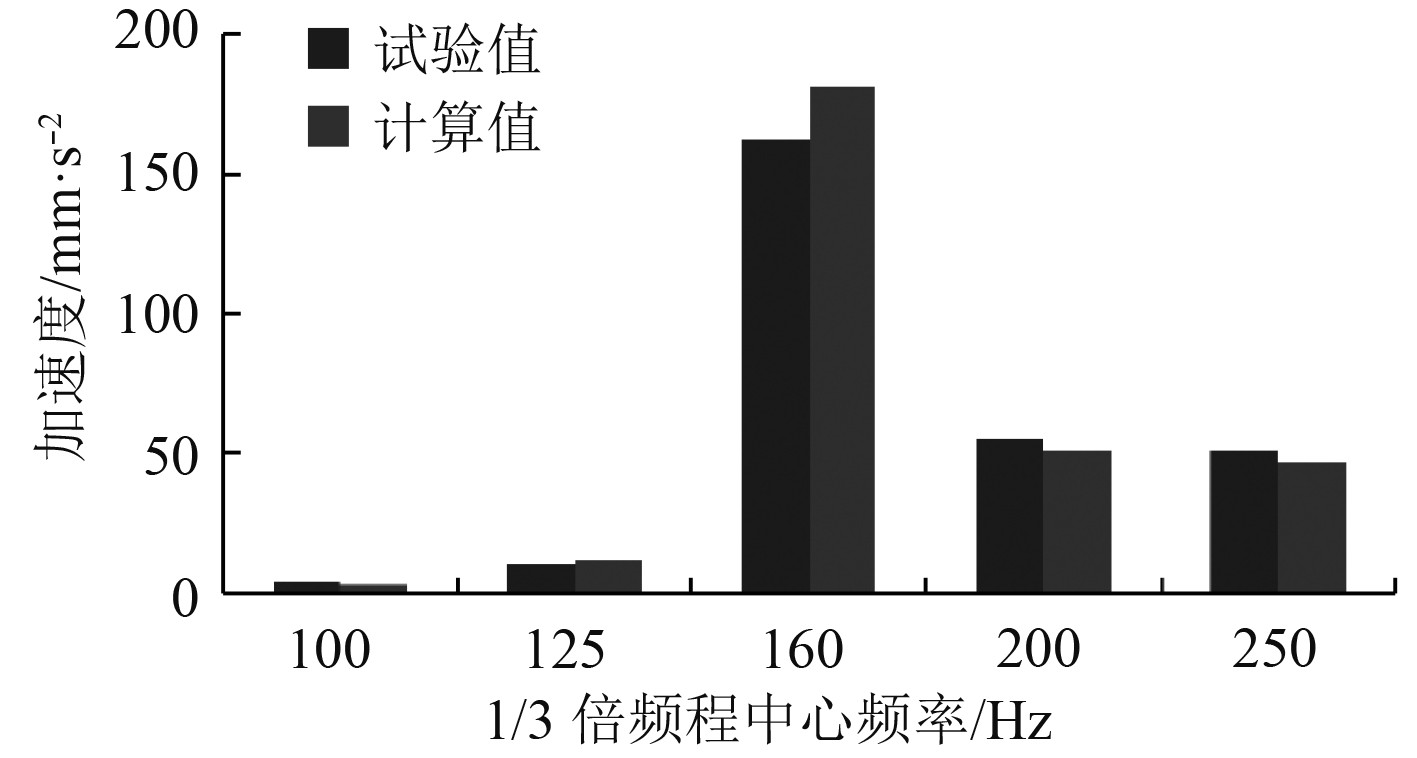图 13 node7 1/3倍频程加速度有效值a1/3 Fig. 13 Acceleration RMS a1/3 of node7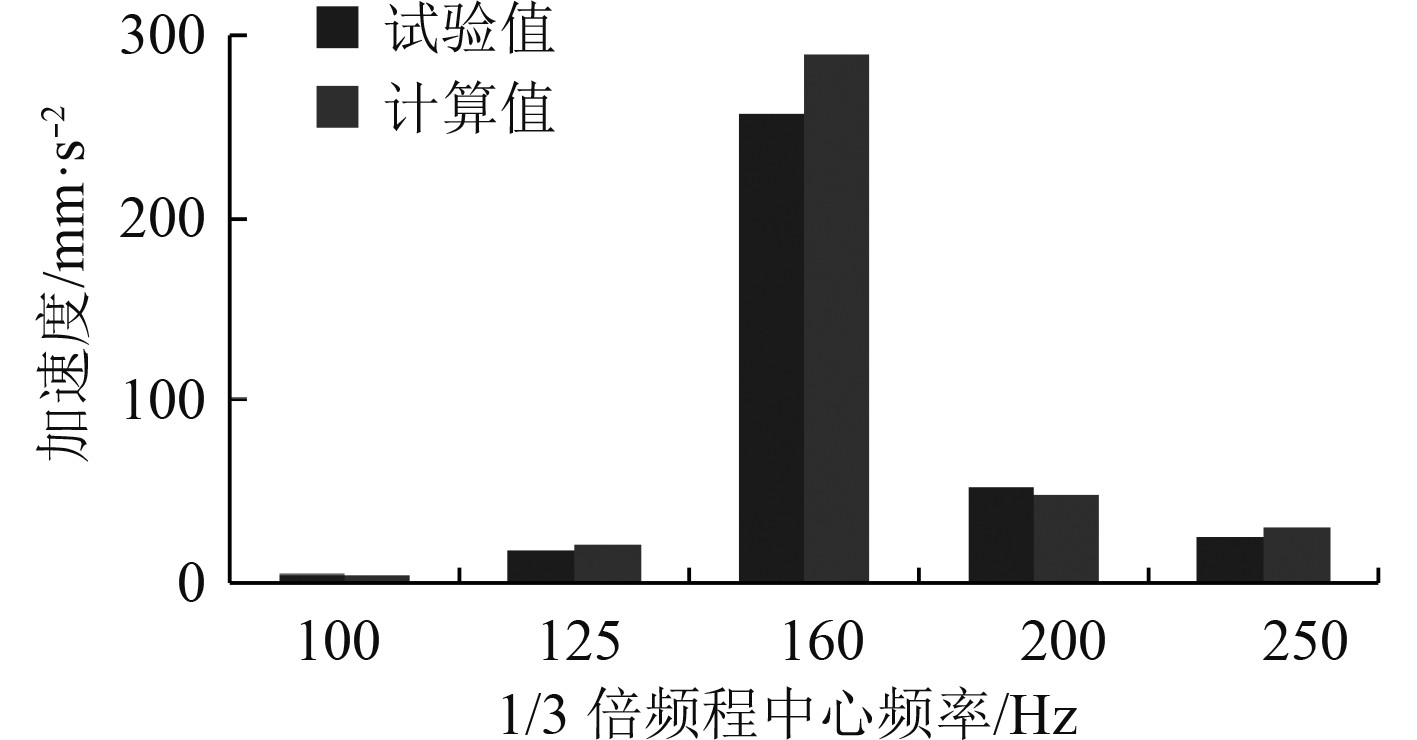图 14 node8 1/3倍频程加速度有效值a1/3 Fig. 14 Acceleration RMS a1/3 of node8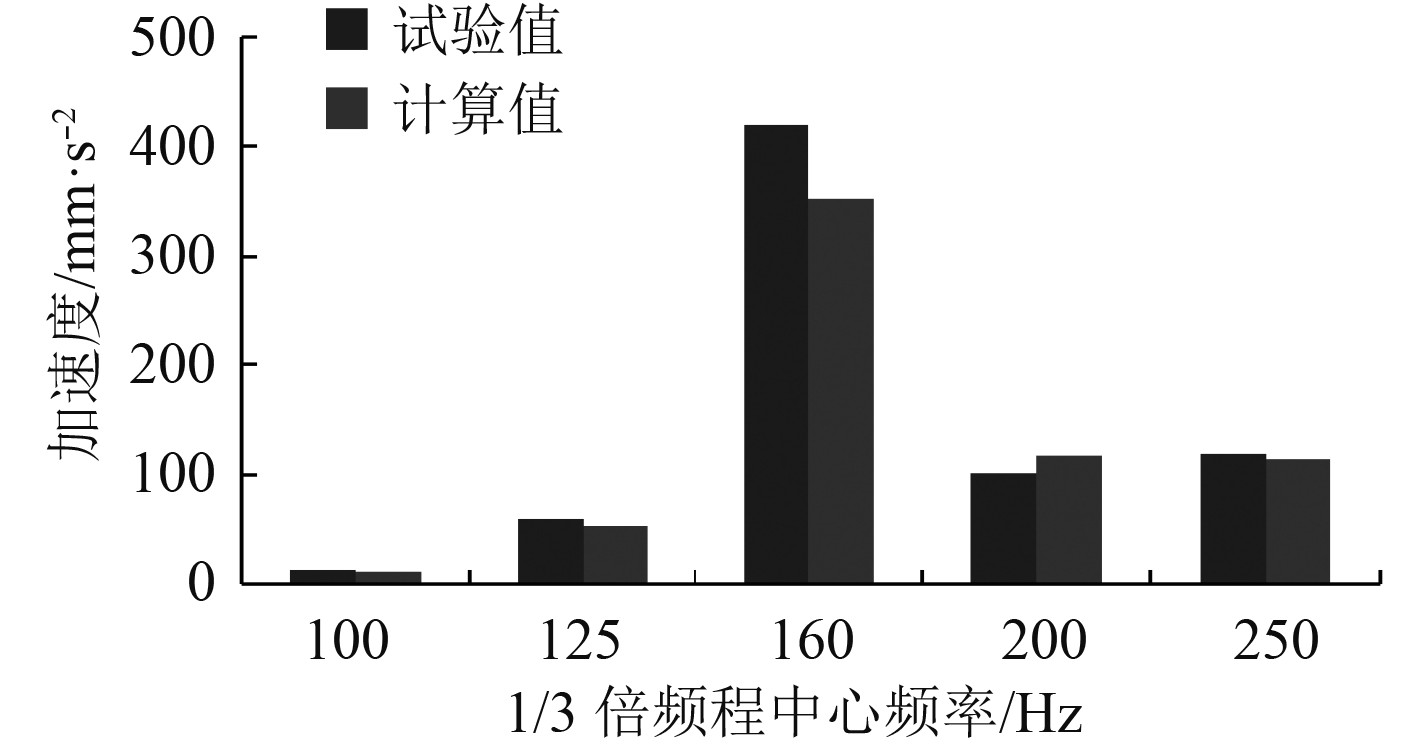图 15 node12 1/3倍频程加速度有效值a1/3 Fig. 15 Acceleration RMS a1/3 of node12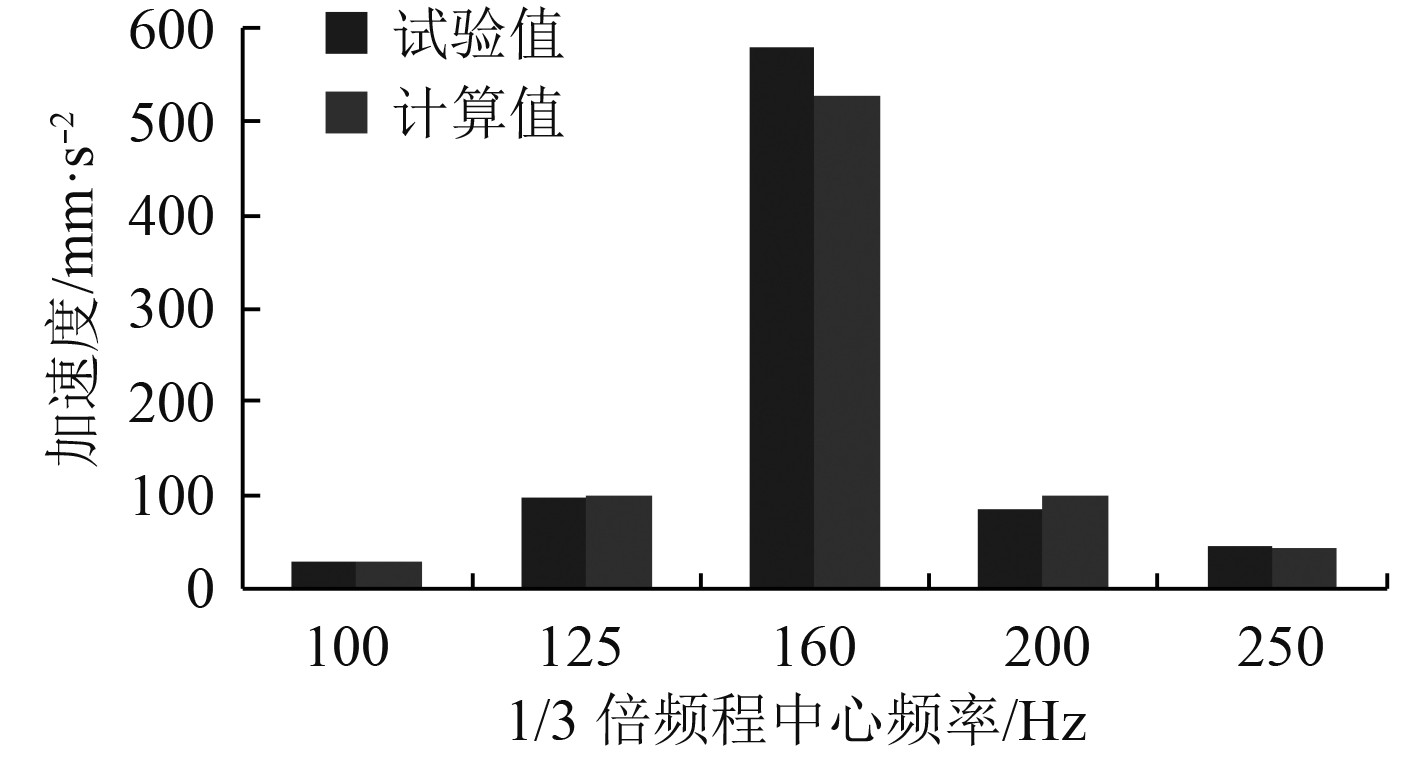图 16 node13 1/3倍频程加速度有效值a1/3 Fig. 16 Acceleration RMS a1/3 of node13

4 结　语

1）利用修正公式计算所得阻尼压筋板模态损耗因子与文献中的试验结果误差很小，验证了该修正公式的正确性。

2）在随机响应计算中可忽略阻尼弹性模量频变与MCK方程刚度矩阵的耦合作用，仅考虑其对模态损耗因子的影响。

3）采用基于修正模态阻尼损耗因子的随机响应计算流程，可以获得较好的数值计算结果，相关修正公式和计算方法可以为阻尼减振的工程应用提供参考。

  周云. 粘弹性阻尼减震结构设计[M]. 武汉: 武汉理工大学, 2006: 116–128.  LU Y P, EVERSTINE G C. More on finite element modeling of damped composite systems[J]. Journal of Sound & Vibration, 1980, 69(2): 199-205.  HU Y C, HUANG S C. The frequency response and damping effect of three-layer thin shell with viscoelastic core[J]. Computers & Structures, 2000, 76(5): 577-591.  IU V P, CHEUNG Y K, LAU S L. Non-linear vibration analysis of multilayer beams by incremental finite elements, Part II: Damping and forced vibrations[J]. Journal of Sound & Vibration, 1985, 100(3): 373-382.  BAGLEY R L, TORVIK P J. On the fractional calculus model of viscoelastic behavior[J]. Journal of Rheology (1978-present), 1986, 30(1): 133-155. DOI:10.1122/1.549887  MCTAVISH D J, HUGHES P C. Modeling of linear viscoelastic space structures[J]. Asme Transactions Journal of Vibration Acoustics, 1993, 115(1): 103-110. DOI:10.1115/1.2930302  JOHNSON C D, KIENHOLZ D A. Finite element prediction of damping in structures with constrained viscoelastic layers[J]. Aiaa Journal, 2012, 20(20): 1284-1290.  CAO X, MLEJNEK H P. Computational prediction and redesign for viscoelastically damped structures[J]. Computer Methods in Applied Mechanics & Engineering, 1995, 125(1-4): 1-16.  邓年春, 邹振祝, 杜华军, 等. 约束阻尼板的有限元动力分析[J]. 振动工程学报, 2003, 16(4): 489-492. DENG Nian-chun, ZOU Zhen-zhu, DU Hua-jun, et al. A finite element dynamic analysis of constrained plates[J]. Journal of Vibration Engineering, 2003, 16(4): 489-492. DOI:10.3969/j.issn.1004-4523.2003.04.021  孙社营. 阻尼压筋板的动态力学性能研究[J]. 噪声与振动控制, 2000(02): 19-23. SUN She-ying. Dynamic investigation of a damped stiffened plate[J]. Noise and Vibration Control, 2000(02): 19-23. DOI:10.3969/j.issn.1006-1355.2000.02.003  RAO D K. Frequency and loss factors of sandwich beams under various boundary conditions[J]. ARCHIVE Journal of Mechanical Engineering Science 1959-1982(vols 1-23), 1978, 20(5): 271-282.  祝驰誉, 温华兵. 丁基橡胶阻尼材料对基座减振的实验研究[J]. 造船技术, 2015(2): 50-53. ZHU Chi-yu, WEN Hua-bin. An experimental study on butyl rubber damping material applied to base structure[J]. Marine Technology, 2015(2): 50-53. DOI:10.3969/j.issn.1000-3878.2015.02.011